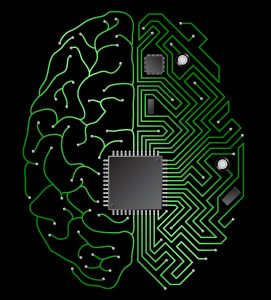# A Solution Architect’s Questions about the Biological Brain

As machine learning evolves in the pursuit of AI, one of the conversations we should ramp up is between ML engineers/researchers (software/hardware) and biologists/neurologists/biochemists (wetware). Such exchange for information could drive both fields of study, each domain serving questions, challenges and working models to the other.

What is the throughput of one synapse, in signals / second?

How can we model one signal? How many bits would it minimally take to represent?

What is the throughput of one synapse, in bits/second?

How can the real activation function be measured on an actual neuron?

• Assuming synapses have weights, how are weights increased or modified? What is the signalling or transmission mechanism for enforcing and respectively dissolving synapses?
• Are there several types of weight functions, depending on the type of neuron?
• Are the weight functions symmetrical on all input (albeit with different weights)?

What is the latency of firing a synapse?

• Time needed for internal firing of source neuron
• Travelling time on axon
• Travelling time in dendrite
• Time needed for internal firing of destination neuron

How do neurons handle the asynchronicity between inputs? Does each input have a expiration (fade off) time?

Does firing of one synapse have side-effects (i.e. short term, long term)? For how long? Examples of side-effects may include:

• Saturation – making it harder for the synapse to fire a (k+1)th time after it fired (k) times

Does firing of one neuron have side-effects? For how long?

What is the distribution (histogram) of the working frequencies for neurons? (measured in Hz or operations per second)

What is the distribution (histogram) of axons by number of outbound neurons/dendrites?

What is the distribution (histogram) of distances travelled by dendrites?

What is the distribution (histrogram) of distance*throughput (outbound) of axons?

What is the distribution (histogram) of distance*throughput (inbound) of dendrites?

What is the distribution (histogram) of number of neurons per brain region?

What is the distribution (histogram) of number of synapses per brain region?

What is the throughput of the human brain? (input-output operations/second)

• Breakdown per activity
• Breakdown per time of day
• Breakdown by area of the brain

What is the processing capacity of the human brain? (computations-operations per second, such as the activation functions)